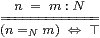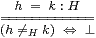## 4  Introducing the calculus

Now we start to present our new calculus, which is called Abstract Stone Duality, in a relatively informal way. For a more formal treatment that is better suited to logicians please see [I] instead. Indeed, readers of both kinds should be aware that the notation in this paper is the result of carefully chosen de-formalisation of the earlier and more foundational work in the ASD programme, in order to make it look more like the usual idioms of mathematics.

The first few axioms are very familiar facts about the real line and other spaces.

Axiom 4.1 In this paper, we take ∅, 1, 2, 3,..., ℕ, ℤ, ℚ, ℝ, I≅[0,1]⊂ℝ and the Sierpiński space Σ as base types. If X and Y are types then so are their product X× Y and sum (disjoint union) X+Y. The open and closed subspaces of X that are defined by a continuous function φ:X→Σ are also types, as is the function-space ΣX. However, we stress that these do not use set theory.

Every variable x or expression a has a type; we write x:X or a:X to say what it is.

Along with X× Y, X+Y and ΣX come projections, pairing, inclusions, case-analysis, function-application and abstraction operations. These are explained in any account of simple type theory, such as [Tay99, §2.3].

You may substitute the phrase “locally compact topological space” for “type” if you wish, just as you understood elements of a group as matrices, and numbers as collections of beads, earlier in your mathematical education. However, our language is intended to be complete in the sense that we can reason in it using just the rules that we state, and not by forever referring back to the model in traditional topology. These rules extend those of arithmetic, and the role of types is to stop us from doing silly things like applying logical operators to numbers or vice versa.

The fundamental place of ℝ in science surely justifies introducing and axiomatising it independently, as we do in this paper. On the other hand, [I] constructed it using two-sided Dedekind cuts of ℚ in the ASD calculus. So you may say that the Axioms about ℝ here were Theorems there, or that that paper implements the ideas of this one, or again that it shows that these axioms provide a conservative extension. However, giving some implementation is not an exclusive action: there may be better ones.

Axiom 4.2 Terms of any type may be defined by recursion over ℕ, for example ℕ itself has addition and multiplication. The objects ℤ, ℚ and ℝ carry the structure of commutative rings, with the usual notation, inclusions and algebraic laws. We shall consider division and general recursion shortly.

Axiom 4.3 The types ℕ, ℤ and ℚ all carry the usual six binary relations

 x=y,   x≠ y,   x< y,   x≤ y,   x> y   and   x≥ y,

but ℝ and I only have

 x≠ y,   x< y  and   x> y.

Notice again that we distinguish ≤ in arithmetic from ⊑ in logic and topology.

Axiom 4.4 These nine expressions are propositions, as are

 ⊤  (true),   ⊥  (false),   σ∧τ,   σ∨τ,    ∃ x:S.φ (x)   and   ∀ x:K.φ (x),

whenever σ, τ and φ(x) are, except that the quantifiers may only be formed when S is overt and K compact, as we shall explain later. Propositions are the same as terms of type Σ. Therefore, for the topological and computational reasons that we gave in the previous section, we cannot use ¬ or ⇒ to form them.

However, such a logic would be too weak to be useful. An important difference between set theory and topology is that one has just a single notion of subset, whilst the other has many different kinds of subspace: open, closed, compact, overt, connected and so on (Section 7). We therefore need to make analogous distinctions in the terminology that we use for the corresponding logical properties.

Definition 4.5 If σ and τ are propositional expressions and a,b:X are any two expressions of the same (but any) type then

 σ⇒τ,       σ⇔τ     and     a=b:X

are called statements. The intended meaning of the first is that the open subspace represented by σ is contained in that represented by τ,  or that if the program σ terminates then so does τ,  whilst the containment between corresponding closed subspaces is the other way round. The second says that they coincide. Since σ⇒τ iff σ∧τ⇔τ,  all three can be seen as equations between terms of type Σ or X, but they are not terms themselves.

We write & instead of ∧ for the conjunction of statements; note that ∃, ∀, ⇒ and ⇔ cannot be used to combine statements into more complicated things (in this version of the calculus, but cf[M]). We shall, however, write

 α⇒β⇔γ⇒δ     for     (α⇒β)  &  (β⇒γ)  &  (γ⇒β)  & (γ⇒δ),

just as we do with equations and inequalities of numbers. We shall see how statements can be used to define subspaces, in particular compact ones, in Sections 7 and 8.

A propositional expression φ x that contains a parameter (free variable) x:X defines a function X→Σ, which is automatically continuous, and so (cf. Proposition 3.9) both an open subspace U and a closed one C, where

 φ x⇔⊤   means   x∈ U     and     φ x⇔⊥   means   x∈ C.

These are statements, although we sometimes write them more briefly as φ x and ¬φ x respectively. Since we are not allowed to write ¬¬φ x or φ x∨¬φ x, the subspaces U and C are not “complementary” in anything like the set-theoretic sense.

We may use statements as axioms, assumptions or conclusions, as we shall explain in the next section.

Now consider the meaning of statements that are formed directly from the propositional arithmetical relations on ℕ and ℝ that we introduced in Axiom 4.3. Recall that we said that ℝ didn’t have =, ≤ or ≥ as propositions, because the subspaces of ℝ×ℝ that these define are not open.

Examples 4.6 For x,y:ℝ, the statements

 (x≠ y)=⇒⊥,      (x> y)=⇒⊥      and     (x< y)=⇒⊥

mean x=y, xy and xy respectively. We augment our notation for statements with these three familiar symbols, but now equality has become overloaded:

Definition 4.7 We call a type N (such as ℕ or ℚ but not ℝ) discrete if the two statements in the rule on the left below are interchangeable. The top one is the statement of equality of two terms (n,m) that we could make for any type, and the bottom one is a statement of equality of two propositions, (n=N m) and ⊤. A discrete space is special in that the proposition (n=N m) of equality is meaningful for it (as it is for ℕ and ℚ but not ℝ) and makes this rule valid.Definition 4.8 Similarly, we call a type H (such as ℕ, ℚ or ℝ) Hausdorff if the statement of equality of terms (h,k) on the top right is interchangeable with the statement of equality of propositions below it, one of which is the proposition (hH k) of inequality. In other constructive accounts of analysis, ≠ is sometimes called apartness and written h#k.

Since propositions provide a logical way of describing open subspaces, these two properties say that the diagonal subspace XX× X is respectively open or closed. In traditional topology, which has arbitrary unions, every discrete space is Hausdorff, but this is not so in ASD: =N may be defined whilst ≠N isn’t (Example 16.5). When they are both defined, as they are for ℕ, they are complementary (Definition 6.4). Lemma 5.9 collects some of the properties of equality and inequality.

We can now formulate some of the basic properties of ℝ as axiomatic statements:

Axiom 4.9 The types ℚ and ℝ are totally ordered Hausdorff fields, i.e

 (x≠ y) ⇔ (x< y)∨(y< x)     and     x−1  is defined iff  x≠ 0.

The order relation < is also transitive and located,

 (x< y)∧(y< z) =⇒ (x< z)  =⇒ (x< y)∨ (y< z).

Beware that the word “located” has several meanings in constructive analysis, which are related but not directly so.